4.12 Balance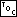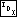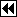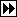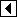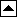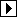Sometimes binary trees can grow to become much taller than their optimum height. For example, the following binary tree was one of the tallest from a sample of 100 15-node trees built by inserting nodes in random order:

The average number of comparisons required to find a random node in this tree is (1 + 2 + (3 * 2) + (4 * 4) + (5 * 4) + 6 + 7 + 8) / 15 = 4.4 comparisons. In contrast, the corresponding optimal binary tree, shown below, requires only (1 + (2 * 2) + (3 * 4) + (4 * 8))/15 = 3.3 comparisons, on average. Moreover, the optimal tree requires a maximum of 4, as opposed to 8, comparisons for any search: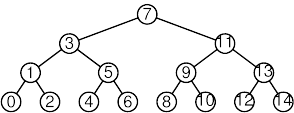Besides this inefficiency in time, trees that grow too tall can cause inefficiency in space, leading to an overflow of the stack in bst_t_next(), bst_copy(), or other functions. For both reasons, it is helpful to have a routine to rearrange a tree to its minimum possible height, that is, to balance (see balance) the tree.

The algorithm we will use for balancing proceeds in two stages. In the first stage, the binary tree is “flattened” into a pathological, linear binary tree, called a “vine.” In the second stage, binary tree structure is restored by repeatedly “compressing” the vine into a minimal-height binary tree.

Here's a top-level view of the balancing function:

```87. <BST balance function 87> =
<BST to vine function 89>
<Vine to balanced BST function 90>

void bst_balance (struct bst_table *tree) {
assert (tree != NULL);

tree_to_vine (tree);
vine_to_tree (tree);
tree->bst_generation++;
}
```

This code is included in 29.

```88. <BST extra function prototypes 88> =

/* Special BST functions. */
void bst_balance (struct bst_table *tree);
```

This code is included in 24, 247, 372, and 486.